Next: Analysis of the Rabin Up: Blair's Cryptography Notes Previous: Terminology   Contents

# A probabilistic test for primality

Suppose we want to test whether 247 is a prime number. Recall two facts about prime numbers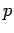:
1.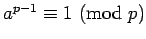if.
2. If, then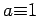or. []
Suppose we randomly chooseand test for consistency with these conditions. Since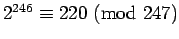we can conclude immediately that 247 is not a prime.

Perhaps we were lucky with. If we try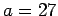, we get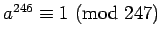. However,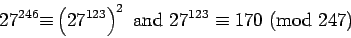which is inconsistent with the second condition, again implying 247 is not a prime.

Not every choice of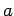is inconsistent with the conditions. For example,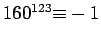(hence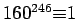) and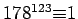. However, the fact that some choices ofgive a proof that a number is not prime suggests the following test:

Rabin's Primality Test. Let, where m is an odd number. Chooseat random. Compute the sequenceThis sequence is consistent withbeing a prime ifor if the sequence has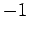at some point, followed by 1 for all subsequent terms. In all other cases,provides a proof thatis not prime (this is usually described by saying thatis a witness thatis not prime). Repeat this test for some number of random choices of, and conclude thatis a prime if none of the chosenis a witness.

Two features of the test should be emphasized. It does not provide an absolute guarantee thatis a prime, only that it is probably a prime (we will analyze exactly how probable in the next section). Secondly, when we knowis not a prime, we do not know what its factors are-- factoring is much more difficult than testing for primality.

[A different probabilistic test is described near the end of the RSA paper.]

SubsectionsNext: Analysis of the Rabin Up: Blair's Cryptography Notes Previous: Terminology   Contents
Translated from LaTeX by Scott Sutherland
2002-12-14